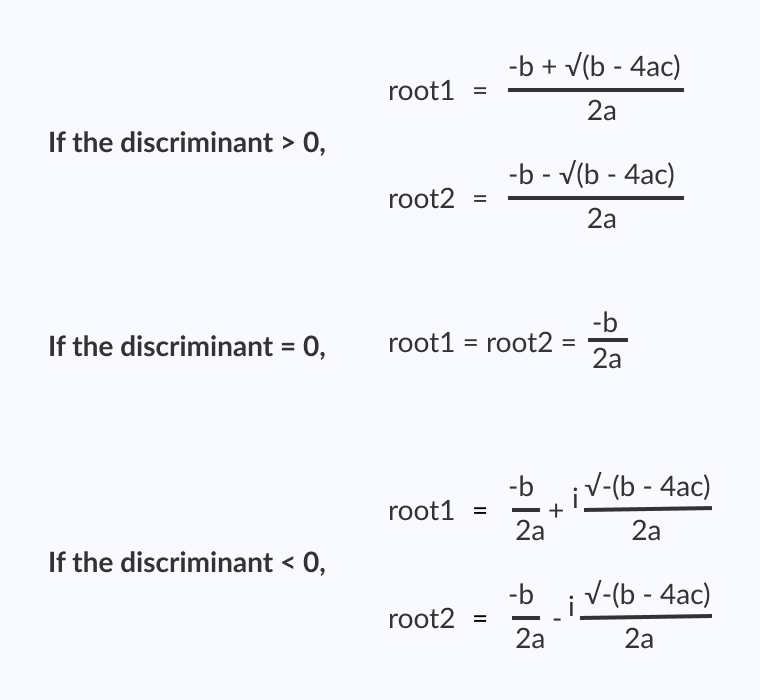# C Program to Find the Roots of a Quadratic Equation

In this example, you will learn to find the roots of a quadratic equation in C programming.

To understand this example, you should have the knowledge of the following C programming topics:

The standard form of a quadratic equation is:

```ax2 + bx + c = 0, where
a, b and c are real numbers and
a != 0
```

The term `b``2``-4ac` is known as the discriminant of a quadratic equation. It tells the nature of the roots.

• If the discriminant is greater than `0`, the roots are real and different.
• If the discriminant is equal to `0`, the roots are real and equal.
• If the discriminant is less than `0`, the roots are complex and different.## Program to Find Roots of a Quadratic Equation

``````#include <math.h>
#include <stdio.h>
int main() {
double a, b, c, discriminant, root1, root2, realPart, imagPart;
printf("Enter coefficients a, b and c: ");
scanf("%lf %lf %lf", &a, &b, &c);

discriminant = b * b - 4 * a * c;

// condition for real and different roots
if (discriminant > 0) {
root1 = (-b + sqrt(discriminant)) / (2 * a);
root2 = (-b - sqrt(discriminant)) / (2 * a);
printf("root1 = %.2lf and root2 = %.2lf", root1, root2);
}

// condition for real and equal roots
else if (discriminant == 0) {
root1 = root2 = -b / (2 * a);
printf("root1 = root2 = %.2lf;", root1);
}

// if roots are not real
else {
realPart = -b / (2 * a);
imagPart = sqrt(-discriminant) / (2 * a);
printf("root1 = %.2lf+%.2lfi and root2 = %.2f-%.2fi", realPart, imagPart, realPart, imagPart);
}

return 0;
}
``````

Output

```Enter coefficients a, b and c: 2.3
4
5.6
root1 = -0.87+1.30i and root2 = -0.87-1.30i
```

In this program, the `sqrt()` library function is used to find the square root of a number. To learn more, visit: sqrt() function.